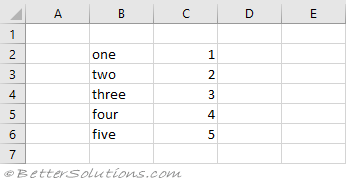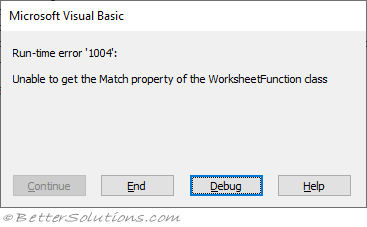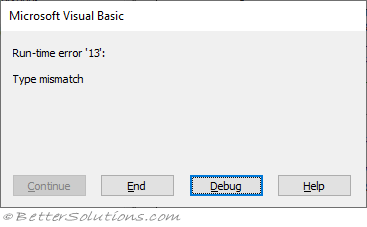# Error Handling

### WorksheetFunction. or Application.

These two lines of code call the built-in Excel MATCH function.

`lookup_result = Application.WorksheetFunction.Match("three", myRange, False) lookup_result = Application.Match("three", myRange, False) `

If the value you are looking for exists then both these lines are equivalent.
But if the value does not exist, then there is a subtle difference between them.### Application.WorksheetFunction.Match

The MATCH function returns the position of a value in a list or cell range.
Here we are searching for the position of the value "three", which does exist.

`Dim lookup_result As Variant Dim myRange As Excel.Range Set myRange = Range("B2:B6") lookup_result = Application.WorksheetFunction.Match("three", myRange, False) Call MsgBox(lookup_result) = 3 `

But what happens if we try and search for the value "seven", which does NOT exist.

`lookup_result = Application.WorksheetFunction.Match("seven", myRange, False) `

We get a run-time error.If we step through the code this error is being returned by the call to the Match function.
This can be fixed by putting this code into its own dedicated function with error handling.
The dedicated function "Excel_MatchFunction" has a return data type of "Variant" so it can return values AND error messages.

`Public Sub Test()    Call MsgBox(Excel_MatchFunction("seven")) = "not found" End Sub Public Function Excel_MatchFunction(ByVal myValue As String) As Variant    Dim lookup_result As Variant    Dim myRange As Excel.Range       On Error GoTo ErrorHandler    Set myRange = Range("B2:B6")    lookup_result = Application.WorksheetFunction.Match(myValue, myRange, False)    Excel_MatchFunction = lookup_result       Exit Function ErrorHandler:    Excel_MatchFunction = "not found" End Function `

### Application.Match

Now lets do exactly the same thing with Application.Match.
Here we are searching for the position of the value "three", which does exist.

`Dim lookup_result As Variant Dim myRange As Excel.Range Set myRange = Range("B2:B6") lookup_result = Application.Match("three", myRange, False) Call MsgBox(lookup_result) = 3 `

But what happens if we try and search for the value "seven", which does NOT exist.

`lookup_result = Application.Match("seven", myRange, False) `

We also get a run-time error, but this error is different.If we step through the code this error is because we are trying to display an Error with a MsgBox.
This can be fixed by converting the lookup result to a string.

`Call MsgBox( VBA.CStr(lookup_result) ) = "Error 2042" `

We do not need to use Error Handling to get around this problem, we can just use the VBA ISERROR function.

`Dim lookup_result As Variant Dim myRange As Excel.Range Set myRange = Range("B2:B6") lookup_result = Application.Match("three", myRange, False) If (VBA.IsError(lookup_result) = True) Then    lookup_result = "not found" End If Call MsgBox(lookup_result) = "not found" `

### Important

Both the VBA.FV function and WorksheetFunction.FV function work.
Both the VBA.REPLACE function and the WorksheetFunction.SUBSTITUTE function work even though they are very similar.